#### You may also like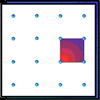### Geoboards

This practical challenge invites you to investigate the different squares you can make on a square geoboard or pegboard.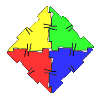### Polydron

This activity investigates how you might make squares and pentominoes from Polydron.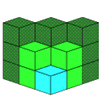If you had 36 cubes, what different cuboids could you make?

# Prompt Cards

##### Age 7 to 11Challenge Level
Ruby W and Ruby F from  Hadnall CE Primary School tried both challenges;

Charlie's Number
"Using clues 2 and 4, we realised that our number had to be 3 digits long. Using clue 3, we initially worked out that our number need to end in 3, 5, 7 or 9. As Charlie's number is palindromic, the third and first digits need to be the same. Therefore, due to rule 5, our first and third digits had to be 3 (to make sure our number is less than 500). Finally, using rule 3, we used 8 as our second digit (to make the difference between the largest and smallest digits 5). We used the rest of the rules to check our number to see if it worked. 383 is a prime number and therefore will only have two divisors (itself and one and NOT 3)."
Tower problem
"Rule one meant we started with a yellow cube on top. We then placed a red cube on top of a green cube and placed this below the yellow - for rule two and part of rule three. We both blue cubes on the outside faces of our green cube - for rule four. Finally, we placed a yellow cube underneath the red to satisfy all the rules. Realising that "above" did not necessarily mean "directly above"/"touching", made this problem a lot easier.
We then tried to create a tower which was a single cube wide. By placing both blue cubes either side of the green cube, we satisfied rule 4. Placing a red cube above a blue cube satisfied rule two. Placing a yellow cube on the top and bottom of the tower satisfied the remaining rules."

James Huggins from Norman Gate School wrote;

Using the information I had, I created different numbers which were over 100 and prime. Then I checked to see whether the difference between the largest digit and smallest digit was 5. I then checked to see which of these numbers had a total of 14, very quickly I could see the answer was likely to be 383. This number has no decimal places and was odd. I tried to divide this number by 3, 7 and 9 to double check it was prime. I knew it wouldn't be divisible by 5 because it didn't end with a 5 or 0. I knew it wasn't divisable by 4, 8, 6 or 2 as it was an odd number. 383 is over 100 and under 500.

Frances and Charlie from Millfields Primary School sent in the following;

We followed the clues one by one and wrote down a number which satisfied that criteria. When we looked at the next clue, we adjusted and changed our numbers; we kept doing this until we reached the last clue. We were amazed that we had found an answer which worked for all the clues. We could not find another number which worked - everyone in our class had a go and
no-one succeeded!

Farha from Arnhem Wharf Primary School wrote;

My starting point was clue 4. Since I knew the sum has to be 14 and from clue 3 the difference had to be 5. I started using random numbers. For example: 626 but the difference here is 4. Then I used 8 and 8-5=3. So far my numbers were 3 and 8. 3+8=11 and I need 14 so I just added 3. At the end my numbers were 383. I've checked 383 with all the clues and it matches them all.

Zahara  also from Arnhem Wharf Primary School wrote;

I started off by listing the prime numbers from 1 to 9: 2, 3, 5 and 7. After I listed the numbers I crossed out the even prime number(s): 2. Clue number one it tells me it should be a palindromic number, so the number at the end should be the first digit as well (and possibly the second). Thanks to clue number five I knew that the number should not be over 500 therefore eliminating 7 and 5. I then knew the number had to be 3_3 or 333 but then realised the difference between the largest and smallest number must be five, from clue 3, and decided it could not be 333. So I did 3+5 which equals 8. I came to the conclusion that the answer was 383 but I wanted to check whether it would add up to 14 (3+3+8), which clue 4 mentions. The

Mrs Morris and Mrs Cave's Year 4 Maths class at Darras Hall First School said;

After reading the clues we split into 4 groups to look at numbers from 100-200, 200-300, 300-400 and 400-500 as we knew that Charlies number was between 100 and 500. We looked for prime numbers first. We quickly realised that Charlie's number couldn't be in the 200s or 400s because it would then end in a 2 or a 4 because it was palindromic and it would then be an even number. We then realised that Charlie's number couldn't be in the 100s because it would then have a 1 in the hundreds and units and in order to add up to 14 the middle digit would have to be 12, which is impossible. We then knew the number had to start and end in a 3, which would mean that the middle digit would have to be an 8 in order to add up to 14. We also knew that the difference between 3 and 8 is 5. After testing all the other criteria we know that Charlie's number is 383!

Claudia and Trudie from  Maltmans Green worked in a  different way.

1.First write down all the palindromic numbers between 100-500
2.Then cross out all of the even numbers on your list
3.With the numbers left, add all the digits (of each number) together and see if they add up to 14.If they don`t them cross them off
4.After, divide the number (which is left) by 3 and see if the answer is a whole number
5. If the answer is not a whole number then that is the answer to the problem

For the 2nd part we also had Daanya and Elodie from Maltmans, here is their work;
ï¿¼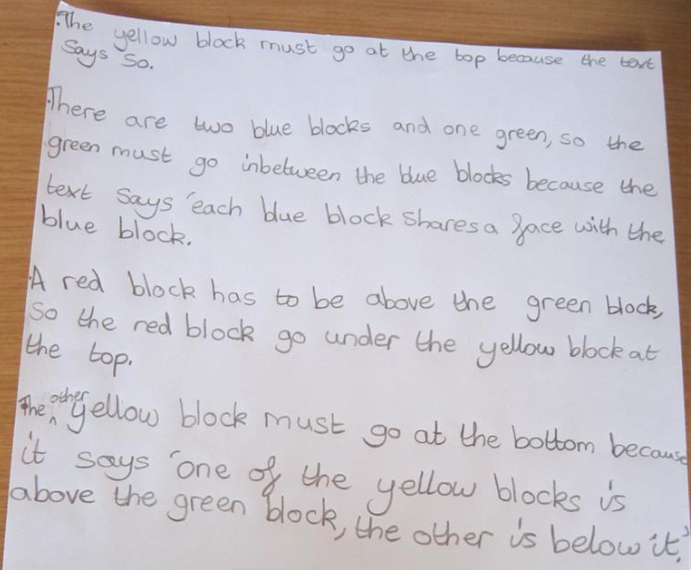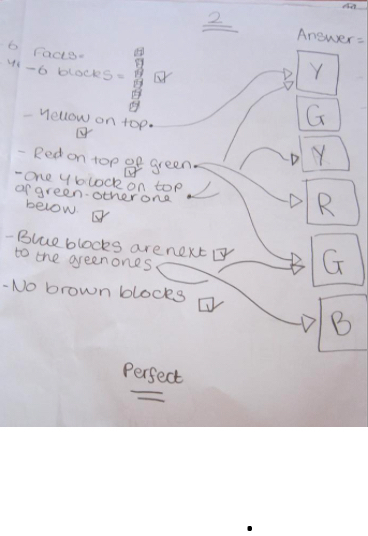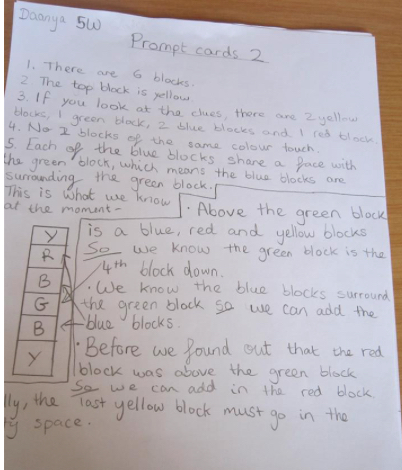Tianyi  from Morley Memorial Primary School wrote;

It is 383.
1) hundred: x; tenth:y. digit: z   2) x+y+z=14,   3) 100   4) Primes 167,239,257,293,383,419,491
5) biggest-smallest = 5  257, 383, 419, 491     6) palindromic     383

Thank you all very much for these different approaches and the excellent explanation of working.